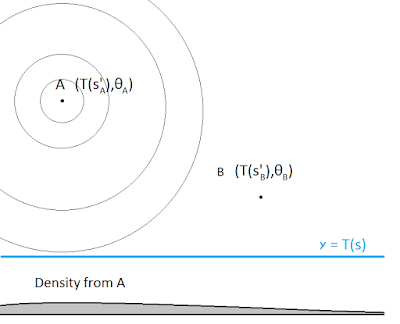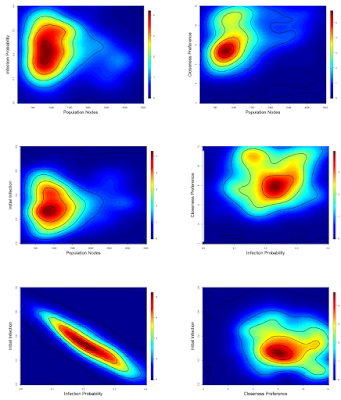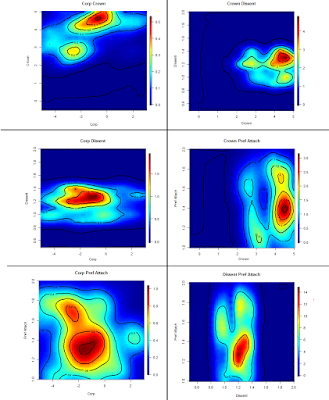## Featured post

### Textbook: Writing for Statistics and Data Science

If you are looking for my textbook Writing for Statistics and Data Science here it is for free in the Open Educational Resource Commons. Wri...

## Friday, 24 June 2016

### Thesis Defence Notes - Approximate Bayesian Computation

These are notes from the slides that were given during my defense. I've clarified some points since then.

The other half of the notes, on the cricket simulator, are found here.

------------------

Approximate Bayesian Computation (ABC) is a method of estimating the distribution of parameters when the likelihood is very difficult to find. This feature makes ABC applicable to a great variety of problems.

The classical ABC method can be summarized as:

Step 1: Select a prior joint distribution F(theta) for the parameters of interest.

Step 2: Observe a sample s from the population you wish to characterize.

Step 3: Compute T(s), a statistic, or set of statistics, from the sample.

Step 4: Repeat the following many times

4A: Generate random parameter values p from F(theta)
4B: Use the values p to simulate a sample s'
4C: Compute the distance between T(s') and T(s), the statistics taken from the simulated and observed samples, respectively.
4D: If the difference is less than some tuning value, epsilon, then accept the values p.

Step 5: The collection of values p from all accepted values is taken as the posterior distribution of the parameters of interest.

Step 6: If the parameters of interest are continuous, a smoothing method is applied to this collection to produce a continuous posterior distribution.

The distance between two statistics can be determined by Manhattan or Euclidean distance, or a weighted distance if deviations between some statistic values are more important than others.

The tuning parameter epsilon determines the balance between accuracy and computational efficiency. A small epsilon will ensure that only generated samples that are very similar to the observed sample will be used. That is, only samples that produce statistics very close to the real sample's statistics. However, many samples will be rejected.

A large epsilon will ensure that many samples will be produced, but some of those samples will have statistics that deviate from the observed sample. Since the process of producing new samples could be time-consuming, depending on the application, this may be a worthwhile trade-off.

Compared to classical Approximate Bayesian Computation method, I made some modifications.

- Instead of having an accept-reject rule, I opted to use every sample but assign weights to the parameters behind each sample based on the distance between T(s') and T(s).

- The weights and smoothing mechanism are determined through a Kernel Density Estimator (KDE)

Specifically, every generated sample is gets treated as a point mass in a space across the statistic values and parameter values.

Consider the example in the following MS-Paint diagrams.

In Figures 1-3, we have a sample with a single statistic value, T(s), vertical, and a single parameter of interest, theta, horizontal. We don't know the parameter value of the observed sample, so we represent the observed sample as a blue horizontal line.

Points A and B represent the statistic and parameter values from each of two generated samples (also A and B, respectively). These are points instead of lines because these samples are simulated using known parameter values.

The gray area at the bottom of each figure is the conditional density of the parameter of the observed sample. The thin lines around points A and B are the contour lines of the probability density, with peaks at the points and decreasing outwards.Figure 1: A generated sample that's far from the observed sample.

In Figure 1, we see the effect of generated sample A on the parameter estimate. The statistic from sample A is far from the statistic of the observed sample, so point A is far from the line. As a result, the probability density does increase or decease much along the line, and therefore the conditional density of the parameter estimate is diffuse, as shown by the shallow gray hill.Figure 2: A generated sample that's close to the observed sample.
In Figure 2, we see sample B's effect. This sample's statistic is close to that of the observed sample. Therefore, point B is close to the line. The conditional density is much steeper around sample B's parameter value. This is because the probability density increases and decreases a lot along the blue line.Figure 3: The combined estimate from both samples.
In Figure 3, we see the cumulative effect of both samples. The conditional density from both resembles that from generated sample B. This is because sample B's effect was given more weight. Generated samples that more closely resemble the observed sample contribute more to the parameter estimate.

I used this method on two applications
1. A theoretical network based on a network of sexual partners in Africa.
2. A real network of precedence citations between decisions of the Supreme Court of Canada.

The theoretical dataset was based on a respondent driven sample. An initial seed of people was selected, presumably by a simple random process. Members of this seed were polled about the number of sexual partners they had in the previous year, their HIV status, and their sexual orientation. For each reported partner, contact information was taken in order to recruit these partners into the sample as well. If the recruitment pool was exhausted before the desired number of respondents, new seed members were found.

Like the basis dataset, we assumed that the sample we were given contained the mentioned covariates of those polled, including the number of partnerships, and we assumed that only the partnerships that led to successful recruitment were available. This implies that large parts of the network could be hidden to us, such as partnerships that 'lead back' into the sample already taken.

Consider these two figures.

Figure 4 is from a sample of a network with identifying information about every reported partnership is given.  Figure 5 is from the same sample, where only the partnerships that led to recruitment are known. Notice that Figure 4 includes lines going out to points that we did not sample directly. Notice also that Figure 4 includes cycles, in which you could go from one point to itself by following the lines and without backtracking.

We don't see these cycles in Figure 5, the recruitment-only network, because a person cannot be recruited if they are already in the sample. A cycle might be a triangle between persons J, K, and L, where all three are connected. Person J can recruit both K and L through those connections, but K cannot recruit L, so we never see the K-L connection. What we see is one person, J, with two distinct partners instead of the whole picture.

Socially, the K-L connection is key information, and mathematically this lack of cycles makes estimation of anything about this network a lot harder.Figure 4: A network with identifying information from the sample.Figure 5: A network with only recruitment information from the sample.

Nevertheless, I used ABC to estimate some features (parameters) of the population that this sample came from.

The parameters of interest were:

- The number of members in the population, (prior: geometric, mean 2000, truncated minimum 400)
- The probability of an HIV infection passing along a partnership, (prior: uniform 0 to 0.3)
- The proportion of this network that was already infected before any of these partnerships (prior: uniform 0 to 0.5)
- The 'closeness preference', a parameter governing the propensity to select partners that are similar to oneself. Zero is no preference, 10 is a strong preference, and a negative number represents a general dislike for those similar to you. (prior: uniform -2 to 10)

I conducted two rounds of Approximate Bayesian Computation by producing 500 and 2500 samples, respectively. The results are here. The yellow and red represent regions of high conditional probability. ABC allows us to narrow down the likely value of the real parameters to these yellow and red regions.

Parameter sets at points all across these graphs were tried, but only those in the non-blue regions produced samples that were anything like the observed sample.Figure 6: Coloured contour maps of conditional densities, social network data.

From these, we can calculate marginal means to get our parameter estimates.

 Parameter True Value Mean (Initial Round) Mean (Final Round) Population Size 1412 2049 2094 Initial Infection Rate 0.467 0.399 0.374 Transmission Chance 0.093 0.175 0.201 Closeness Preference 7.298 6.753 9.352

The second application was on the precedence citations of decisions in the Supreme Court of Canada (SCC). Because this is real data, I am not able to find the ground truth with which to compare my results, but I can showcase how the method behaves with real data.

When this data was collected, there were 10623 documents produced by the SCC, 9847 of which are cases (e.g. disputes between two parties). These decisions are among the more than 3,000,000 cases and other documents recorded in the Canadian Legal Information Institute (CanLII). Figure 7 shows part of the network of SCC cases.Figure 7: Induced subgraph of cases and citations in Supreme Court of Canada

Cases span from 1876 to 2016, with more recent cases represented as points near the top. The larger points are those that have been cited often in Canadian law. Lines between points represent citations between SCC cases. Only 1000 cases are shown here, so the true network between SCC cases has roughly 10 times as many nodes and 100 times as many links.

My general interest in this dataset is to identify the features that make a law prone to being obscure over a long time.

I modelled each SCC case as 'vendor' that was trying to 'sell' citations to future Canadian legal cases. Each SCC case had an attractiveness a, where

and where

βirrel : Parameter determining the decay in attractiveness over time (per 5 years without a citation).
βpa : Parameter determining a preferential attachment factor, assuming that a case becomes more well-known whenever it is cited.
βcorp , βcrown , βdis : Parameters determining increased/decreased attractiveness based on whether a case involved a corporation, the crown, or dissent between Supreme Court Judges, respectively.

what I found was less clear that in was in the synthetic data case, as shown in Figure 8.Figure 8: Coloured contour maps of conditional densities, Supreme Court of Canada data.

It's impossible to read the variable names in this figure, I'm afraid. The jist, however, is that...

- a case that is not cited in five or more is less and less likely to receive citations over time.
- a case that is cited often is more likely to receive citations in the next five years.
- a case that involves a corporation is less likely to be cited.
- a case that involves the crown is more likely to be cited.
- a case that had dissenting opinions by some of the judges is more likely to be cited.